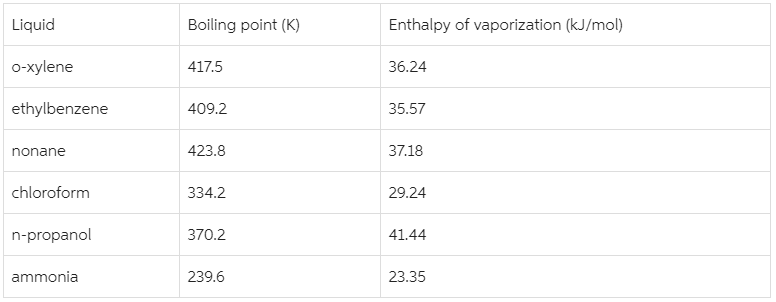# Problem: Predict which of the following compounds follow Trouton's rule:

###### FREE Expert Solution
80% (342 ratings)
###### FREE Expert Solution

We have to determine which of the liquids follow Trouton’s rule.

Trouton’s rule states that the entropy of vaporization for many liquids is in the range of 85-88 J/mol•K.

To find out which of the given liquids follow Trouton’s rule, we will calculate the entropy of vaporization for each of the liquid using the given enthalpy and boiling boiling.

We will use this formula:80% (342 ratings)###### Problem Details

Predict which of the following compounds follow Trouton's rule: